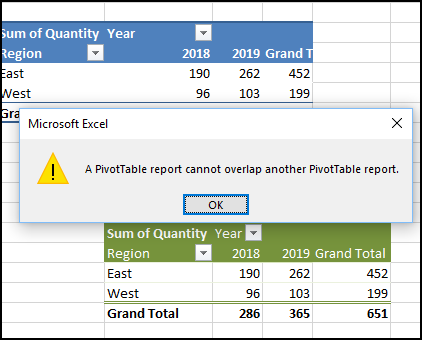## Excel Pivot Table Troubleshooting

If you have a big Excel file, with lots of pivot tables and their source data, I’ve written a macro that might help you. The macros creates a detailed list of all the pivot tables in the workbook, so it’s useful for documentation, and some types of troubleshooting.

### Macro Lists Pivot Table Details

Here’s a screen shot of the first few columns in the pivot table details list that the macro creates.

It lists the sheet name, how many pivot tables are on the sheet, the pivot table names, and a hyperlinked range, where the pivot table is located.Columns E and F show if there are other pivot tables in the same columns or rows. If the pivot tables are too close together, you could end up with overlap error messages.### Source Data Information

For pivot tables that are based on Excel worksheet data (not in the Data Model), the list shows information about the source data too.

You can see the number of records, the column count in the source data, and the count of heading cells that contain text.In column L (Head Fix), an “X” appears if the number of headings doesn’t match the number of columns. That can help you find and fix “field name not valid” problems.### Video: Pivot Table Details Macro

This video shows how the macro helps with trouble shooting, and you can get the sample file from my Contextures website, to follow along.

### The Macro Code

Here is the code for the Pivot Table Details List macro. You can copy it to a regular code module in your workbook, then run it when needed.

```Sub ListWbPTsDetails()
Dim ws As Worksheet
Dim wsSD As Worksheet
Dim lstSD As ListObject
Dim pt As PivotTable
Dim rngPT As Range
Dim wsPL As Worksheet
Dim rngSD As Range
Dim pt2 As PivotTable
Dim rngPT2 As Range
Dim rCols As Range
Dim rRows As Range
Dim RowPL As Long
Dim RptCols As Long
Dim SDCols As Long
Dim lBang As Long
Dim nm As Name
Dim strSD As String
Dim strRefRC As String
Dim strRef As String
Dim strWS As String
Dim strFix As String
Dim lRowsInt As Long
Dim lColsInt As Long
Dim CountPT As Long
On Error Resume Next

RptCols = 13
RowPL = 2

For Each ws In ActiveWorkbook.Worksheets
For Each pt In ws.PivotTables
CountPT = CountPT + 1
If CountPT > 0 Then Exit For
Next pt
If CountPT > 0 Then Exit For
Next ws

If CountPT = 0 Then
MsgBox "No pivot tables in this workbook"
GoTo exitHandler
End If

With wsPL
.Range(.Cells(1, 1), .Cells(1, RptCols)).Value _
= Array("Worksheet", _
"Ws PTs", _
"PT Name", _
"PT Range", _
"PTs Same Rows", _
"PTs Same Cols", _
"PivotCache", _
"Source Data", _
"Records", _
"Data Cols", _
"Refreshed")
End With

For Each ws In ActiveWorkbook.Worksheets
For Each pt In ws.PivotTables
lRowsInt = 0
lColsInt = 0
Set rngPT = pt.TableRange2

For Each pt2 In ws.PivotTables
If pt2.Name <> pt.Name Then
Set rngPT2 = pt2.TableRange2
Set rRows = Intersect(rngPT.Rows.EntireRow, _
rngPT2.Rows.EntireRow)
If Not rRows Is Nothing Then
lRowsInt = lRowsInt + 1
End If
Set rCols = Intersect(rngPT.Columns.EntireColumn, _
rngPT2.Columns.EntireColumn)
If Not rCols Is Nothing Then
lColsInt = lColsInt + 1
End If
End If
Next pt2

If pt.PivotCache.SourceType = 1 Then  'xlDatabase
Set nm = Nothing
strSD = ""
strFix = ""
SDCols = 0
Set lstSD = Nothing

strSD = pt.SourceData

'worksheet range?
lBang = InStr(1, strSD, "!")
If lBang > 0 Then
strWS = Left(strSD, lBang - 1)
strRefRC = Right(strSD, Len(strSD) - lBang)
strRef = Application.ConvertFormula( _
strRefRC, xlR1C1, xlA1)
Set rngSD = Worksheets(strWS).Range(strRef)
SDCols = rngSD.Columns.Count
End If

'named range?
Set nm = ThisWorkbook.Names(strSD)
If Not nm Is Nothing Then
SDCols = nm.RefersToRange.Columns.Count
End If

'list object?
For Each wsSD In ActiveWorkbook.Worksheets
Set lstSD = wsSD.ListObjects(strSD)
If Not lstSD Is Nothing Then
SDCols = lstSD.Range.Columns.Count
End If
Next
End If

If SDCols <> SDHead Then strFix = "X"
With wsPL
.Range(.Cells(RowPL, 1), _
.Cells(RowPL, RptCols)).Value _
= Array(ws.Name, _
ws.PivotTables.Count, _
pt.Name, _
lRowsInt, _
lColsInt, _
pt.CacheIndex, _
pt.SourceData, _
pt.PivotCache.RecordCount, _
SDCols, _
strFix, _
pt.PivotCache.RefreshDate)
Anchor:=.Cells(RowPL, 4), _
End With

RowPL = RowPL + 1
Next pt
Next ws

With wsPL
.Rows(1).Font.Bold = True
.Range(.Cells(1, 1), .Cells(1, RptCols)) _
.EntireColumn.AutoFit
End With

exitHandler:
Set wsPL = Nothing
Set ws = Nothing
Set pt = Nothing
Exit Sub

End Sub```

### Get the Pivot Table Macro Workbook

Get the sample workbook, with the troubleshooting macro, from the Excel Pivot Table List Macros page on my website.

The zipped file is in xlsm format, and contains macros. Enable macros when you open the workbook, if you want to test the code.

______________________

### Pivot Table Details List Macro______________________